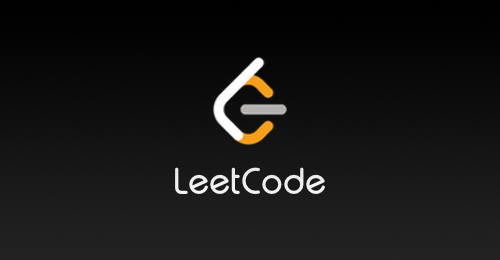# LeetCode 题解：633. 平方数之和，枚举，JavaScript，详细注释

• 2023-02-23
福建
• 本文字数：330 字

阅读完需：约 1 分钟1. 我们可以先枚举a，它的值范围为0 ~ Math.sqrt(c)

2. 同时查找每个a相应的b，如果b为整数，则表示找到两个整数。

3. 如果枚举完了所有可能的a，还未找到b，表示没有找到。

/** * @param {number} c * @return {boolean} */var judgeSquareSum = function (c) {  // 在极限情况下，a^2 + 0 = c，因此a的最大值即为Math.sqrt(c)  let d = Math.sqrt(c)  // a的值可以从0到d，并且是整数  for (let a = 0; a <= d; a++) {    // 计算b的值    let b = Math.sqrt(c - a ** 2)    // 如果b为整数，则表示找到了a和b    if (b === Math.floor(b)) {      return true    }  }  // 退出循环，表示没有找到a和b  return false}## 评论